Select Page

# Disk Scheduling Algorithms

OS use List of Disk Scheduling Algorithms i.e. (FCFS, SSTF, SCAN) for fast access time and Highly throughput. Before to explain disk scheduling, we must know the followings,

As we know, every process requires two types of time for its execution.

```i. CPU time: Execution of process on CPU
ii. I/O time: Time to access the input or output device```

As Hard disk is Input and output device because CPU can read and write the data on it. So, Process request to OS to access the hard disk. This time to access the hard disk is called I/O time.

The method through which an OS decide, which request will be execute next is called disk scheduling.

Let’s explain some important points related to disk scheduling.

### i. Seek Time

Time at which the disk arm arrive at the specific track where the read/write request will be satisfied is known as seek time

### ii. Rotational Latency

Time at which the desired sector comes under the R/W head by rotating itself is called rotational latency. Then Read/write heads can Read or write the data at that specific sector.

### iii. Transfer Time

After accessing the required data from specific sector, it is required to transfer it to CPU for execution. So, the time require to transfer the data from hard disk to CPU is called transfer time.

### iv. Disk Access Time

We can calculate Disk access time through the following equation,

Total Disk_Access_Time = Seek_Time + Rotational_Latency + Transfer_Time

### v. Disk Response Time

It is the average time, spends by each request, waiting for the I/O operation.

## Goals of Disk Scheduling Algorithms

• Fairness
• High throughout
• Minimal traveling R/W head time

## List of Disk Scheduling Algorithms

There are several disks scheduling algorithm where each algorithm has some advantages and disadvantages. We will explain all of the following

• FCFS scheduling algorithm
• SSTF (shortest seek time first) algorithm
• SCAN scheduling
• C-SCAN scheduling
• LOOK Scheduling
• C-LOOK scheduling

### i. FCFS Scheduling Algorithm

In FCFS scheduling algorithm, R/W head of disk, executes the requests  in the same order as they arrive by moving inward and outward of of the disk.

• Simplest algorithm and easy to implement
• There is no starvation in FCFS because every request is serviced as it arrives.

• The scheme does not focus on the seek time.
• Due inappropriate movement of the head, seek time may be very high.

Example

Suppose the request queue sequence is 82, 170, 43, 140, 24, 16, 190 respectively, for a disk with 200 tracks.

Consider the position of R/W Head pointer is 50. Calculate the number of R/W head movements in cylinders, using FCFS scheduling.

Solution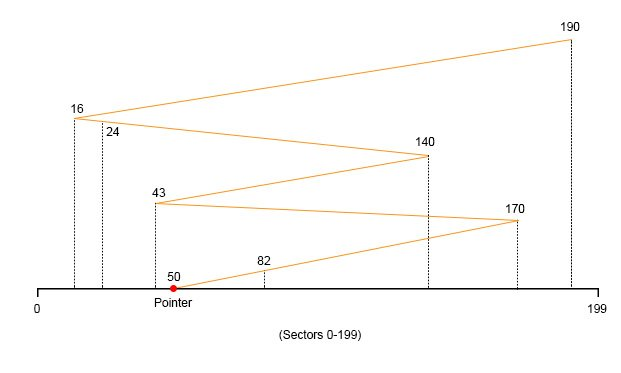Total Number of tracks/cylinders moved by the R/W head is

```= (82-50)+(170-82)+(170-43)+(140-43)+(140-24)+(24-16)+(190-16)
= 32 + 88 + 127 + 97 + 116 + 8 + 174
= 642```

### ii. SSTF Scheduling Algorithms

According to this algorithm, select a request from queue which has the least movement of R/W Head from its current position. This movement of R/W head has no concern with direction, either clockwise or anti clockwise.

• Seek time is reduced
• Highly responsive

• Starvation for some requests may happen.
• Overhead of OS is increased because each time OS has to find the nearest value from disk arm.

Example

```Request queue sequence = 82, 170, 43, 140, 24, 16, 190
Disk Tracks =200
R/W Head pointer is at 50.
Calculate the number of R/W head movements in cylinders, using SSTF scheduling?```

Solution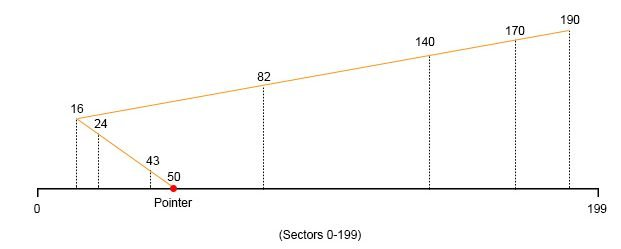`Total Number of tracks/cylinders moved by the R/W head is`
```= (50-43)+(43-24)+(24-16)+(82-16)+(140-82)+(170-140)+(190-170)
= 7 + 9 + 8 + 66 + 58 + 30 + 20
= 208```

### iii. SCAN Algorithm

In this algorithm, the R/W head moves in a particular direction till the end, by executing all the disk requests coming in this direction, and then it turns back and moves in the backward direction by executing the requests coming in that path.

It is also known as Elevator Algorithm because in working, elevator moves in a particular direction completely (till the last floor) and then moves back.

Logic behind the moving of disk arm in a particular direction till the end is, at run time any disk request can happen.

Example

```Request queue sequence = 82, 170, 43, 140, 24, 16, 190
Disk Tracks =200
R/W Head pointer is at 50.
Calculate the number of R/W head movements in cylinders, using SCAN scheduling?
Total Number of tracks/cylinders moved by the R/W head is```

Solution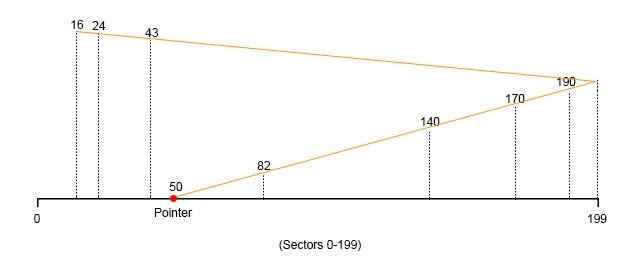`Total Number of tracks/cylinders moved by the R/W head is`
```= (82-50)+(140-82)+(170-140)+(190-170)+(199-190)+(199-43)+(43-24)+(24-16)
= 32 + 58 + 30 + 20 + 9 + 156 +19 +8
= 332```

### iv. C-SCAN algorithm

In C-SCAN algorithm, the R/W head of disk arm moves toward the particular direction by executing the receiving disk requests until it reaches the last track, then it jumps back to the start of sector of the opposite direction without executing any disk request then it  again start moving in that direction by executing the remaining requests.

Example

```Request queue sequence = 82, 170, 43, 140, 24, 16, 190
Disk Tracks =200
R/W Head pointer is at 50.
Calculate the number of R/W head movements in cylinders, using SSTF scheduling?
By considering Direction is toward large value```
```Solution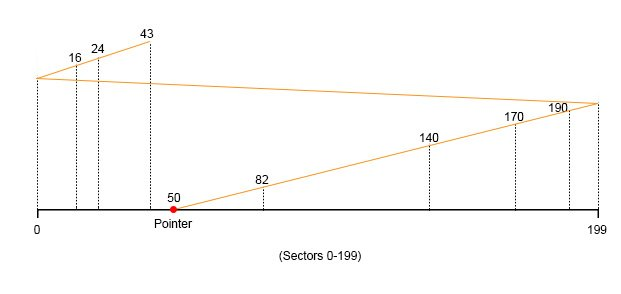Total Number of tracks/cylinders moved by the R/W head is```
```= (82-50)+(140-82)+(170-140)+(190-170)+(199-190) + (199-0) +(16-0) +(24-16) +(43-24)
= 32 + 58 + 30 + 20 + 9 + 199 +16 +8 + 19
= 391```

### v. Look Scheduling

It is just like the SCAN Algorithm but the main difference is, In LOOK scheduling algorithm,  the disk arm stops moving inwards or outwards when there is no more disk accessing request exists in that direction.

Example

```Request queue sequence = 82, 170, 43, 140, 24, 16, 190
Disk Tracks =200
R/W Head pointer is at 50.
Calculate the number of R/W head movements in cylinders, using SSTF scheduling?
By considering Direction is toward large value```
```Solution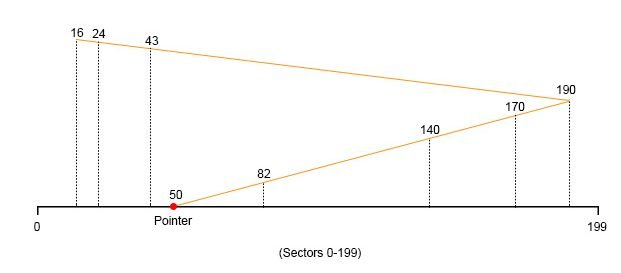Total Number of tracks/cylinders moved by the R/W head is```
```= (82-50)+(140-82)+(170-140)+(190-170) +(190-43)+(43-24)+(24-16)
= 32 + 58 + 30 + 20 + 147 +19 +8
= 314```

### vi. C Look Scheduling

According to C-LOOK algorithm, the arm of the disk moves to outwards of the disk by servicing disk accessing requests until it reaches the highest disk-request track, then it jumps back to the lowest request track without executing any disk-request then again disk-arm starts moving outwards by executing the remaining disk-requests.

Example

```Request queue sequence = 82, 170, 43, 140, 24, 16, 190
Disk Tracks =200
R/W Head pointer is at 50.
Calculate the number of R/W head movements in cylinders, using SSTF scheduling?
By considering Direction is toward large value```
```Solution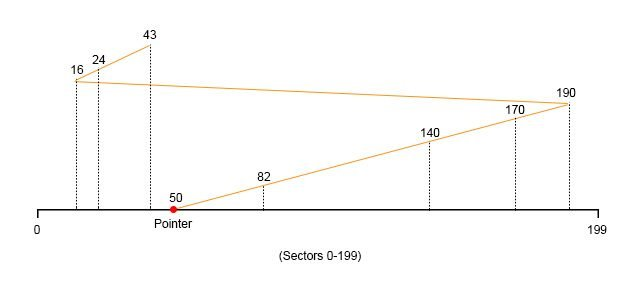Total Number of tracks/cylinders moved by the R/W head is```
```= (82-50) + (140-82) + (170-140) + (190-170) + (190-16) + (24-16) + (43-24)
= 32 + 58 + 30 + 20 + 174 +8 + 19
= 341```

Note: If the B part of this question is

B). If R/W head takes 1ns to move from 1 track to another then total time taken…….?

Solution

Total time taken by R/W head to move from one sector to another = time for 1 sector * total sectors moved                                                                                                                      = 1ns*642= 642ns. (For FCFS)

Help Other’s By Sharing…